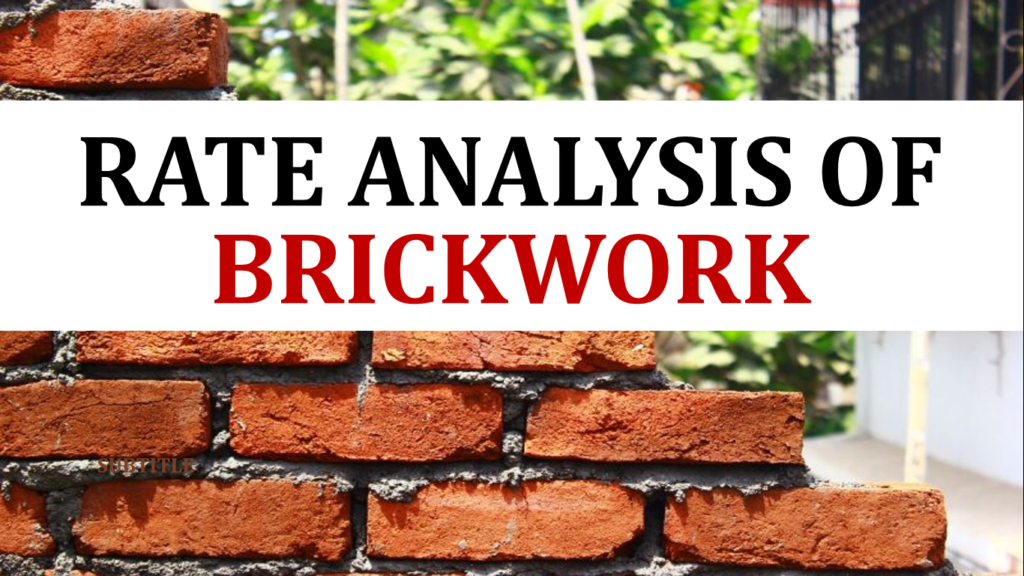Breaking News
Home / QA/QC Engineering / Rate Analysis of Brickwork And Brick Masonry

# Rate Analysis of Brickwork And Brick Masonry

Here we discuss the rate analysis of the brickwork or brick masonry in the superstructure brick size.

The standard size of a brick is 19cm by 9cm by 9cm without mortar.

The standard size of brick is increased 1cm with mortar, so 20cm by 10cm by 10cm.

#### Problem

Find the rate analysis of first-class brickwork in cement mortar where the ratio is 1 to 6 in superstructure with the standard size of brick as above (19cm x 9cm x 9cm)

#### Solution

Brick Size without mortar = 19cm x 9cm x 9cm
In meters, this is 0.19m x 0.09m x 0.09 m
With mortar, the size of brick is increased by 1cm = 20cm x 10cm x 10cm
In meters, this is 0.2m x 0.1m x 0.1m
Let’s consider 10 m3 of volume of brickwork.

### Thumb Rules For Civil Engineers In Building Construction

#### Step 1: Quantity of Materials Calculation

A) Volume of Brick Without Mortar = 0.19 m x 0.09 m x 0.09 m = 0.001539 = 1.539 x 10-3 m3
B) Volume of Brick With Mortar = 0.2 x 0.1 x 0.1 = 0.002 = 2 x 10-3 m3
C) We now need to find out the Quantity of Each Material:

Brick quantity without wastage = 10 divided by volume of brick with mortar = 10 / (2 x 10-3) = 5000 Bricks
Add 5% for brick wastage = 5/100 x 5000 = 250 bricks
Total number of bricks including wastage = 5250 bricks

Wet volume of mortar = 10 – 5000 x 1.539 x 10-3 = 2.31 m3
Add 25% mortar for frog filling (brick indentations) and wastage = 25/100 x 2.31 = 0.577 m3
Adding these two amounts gives us the Total Wet Volume of the Mortar which is 2.9 m3

Next is the Dry Volume of Mortar which is 30% more than the above total wet volume of the mortar = 1.30 x 2.9 = 3.77 m3

Quantity of Cement = ( 3.77 / (1 + 6) ) x 1 = 0.538 m3

Number of Bags of Cement = quantity of cement divided by volume (Volume of 1 bag of cement = 0.035 or 35 litres) = 0.538 / 0.035 = 15.37 (so 16 bags of cement)

Quantity of Sand = ( 3.77 / (1 + 6) ) x 6 = 3.23 m3

#### Step 2: Example Cost Calculation

Particulars Quantity Rate Per Cost
Materials, Cement, Sand, Bricks 16 for cement, 3.23 for sand, 5250 for bricks 350 for cement, 1350 for sand, 4500 for bricks Kg for cement, M3for sand, 1000 numbers for bricks 5600 for cement, 4360.5 for sand, 23625 for bricks
Total Amount 33585.5
Labor
Head mason ½ numbers 600 Per day 300
Mason 10 numbers 500 Per day 5000
Worker A 10 numbers 300 Per day 3000
Worker B 10 numbers 250 Per day 2500
Other 2 200 Per day 400
Total Amount 11200
Total Cost (Materials + Labor) 44785.5

Add 1.5% for water cost = 1.5/100 x 44785.5 = 671.8
Add 1.5% for tools and plants = 1.5/100 x 44785.5 = 671.8
Add 3% for lumpsum = 3/100 x 44785.5 = 1343.6
Add 10% for contractor’s profit = 10/100 x 44785.5 = 4478.55

Add these four to the total = Grand Total = 51951.3

Rate per m3 = 51951.3/10 = 5195.13### THANKS.